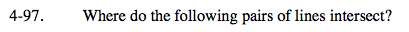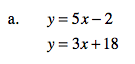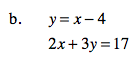### Home > A2C > Chapter 4 > Lesson 4.2.2 > Problem4-97

4-97.
1. Where do the following pairs of lines intersect? Homework Help ✎

1. y = 5x − 2
y = 3x + 18

2. y = x − 4
2x + 3y = 17Refer to the Check Point problems for solving systems of equations.

(10, 48)See part (a).